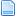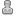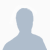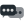Hello Guest it is June 13, 2021, 11:44:25 PM

###Author Topic: parabolic equasion in axis formulas to correct deflection?  (Read 4447 times)

0 Members and 1 Guest are viewing this topic.

####abhi•24##### parabolic equasion in axis formulas to correct deflection?
« on: July 13, 2012, 02:26:35 AM »
Hi,

Ive been doing lots of tests/measurements to correct machine geometry. Both my x and y slump in the middle due to gantry weight and the z also gradually rotates around the y axis apexing in the middle. My linear slides are round bar, for the moment I cant afford to change or add rigidity to the arraignment so I put my thinking cap on and it occurred to me that I could correct these curved looking problems with a parabolic equation. I don't have to correct by much but enough for things to not fit together very well at all.

the standard equation looks like this, so I still have to alter it to get the arc I'm looking for.
Y=(x-a) 2 +b

where a and b are the apex offset of the deflection the "a" term is also has to be inverted (+/-) to give proper cartesian placement.
I haven't tried this yet but wanted to know if there is any reason why it wouldn't or I shouldn't.

####abhi•24##### Re: parabolic equasion in axis formulas to correct deflection?
« Reply #1 on: July 14, 2012, 06:46:43 AM »
hi,
ive ditched using the quadratc equasion in favour of bezier as it has discreat end locations ect. now the triky bit, where or how it would be best implimented. Initialy i was looking at axis formulas but im to understand that they only transform end points, while not being a deal breaker,  it does seem to exclude arc moves which can fave large moves between end points. Im going to look at brains but have read that it doesnt typicaly do that sort of opperation. are there any other places i can perform this sort of opperation.

the only other way i can think to do this sort of thing is to reprocess the nc file and split up the arcs into short splines. maybe first suppliing the work offset so it works properly.

sorry just thinking out loud####Tweakie.CNC••8,725
• Super Kitty##### Re: parabolic equasion in axis formulas to correct deflection?
« Reply #2 on: July 14, 2012, 07:58:01 AM »
Hi Abhi,

It occurs to me that any axis 'slump' you have would vary dependant upon tool loading and not just the axis position so the only realistic solution would be to change to fully supported rails despite the cost.

Just a thought.

Tweakie.
KEEP SAFE !

####Ya-Nvr-No•992##### Re: parabolic equasion in axis formulas to correct deflection?
« Reply #3 on: July 14, 2012, 09:20:21 AM »
I would think first you have to base your calculations on machine home not on part home and then always program all part positions from there. Would be possible but a PITA but I suppose worth a shot. Damn confusing when you look at the Z dro and it is somewhere other than what you programmed because of the formula. Have fun, but Id take Tweaks advice.

####abhi•24##### Re: parabolic equasion in axis formulas to correct deflection?
« Reply #4 on: July 14, 2012, 10:49:47 AM »
changing the mechanical configuration is definately on the agenda, but for the moment i must solve with available resourse. i can strategise the cuts for final pass to be light and the heavy cuts to leave enough stock to absorb the deflection. As im cutting wood and plastic the loads arent huge, but that strategy wont work if i cant achive accuracy under no load. Its a hack for sure, but that is what is availableand what ever i can work out is going to be a whole lot better than what i have, it wont be perfect but it will be improved.

i wonder about the dro thing, how hard would it be to feed post formula values some where else useing brains? im sure something is possible.

####abhi•24##### Re: parabolic equasion in axis formulas to correct deflection?
« Reply #5 on: July 14, 2012, 11:12:16 AM »
oh, yeah im still very much getting used to mach's coordinate environment so im still shooting off in odd directions. My back ground is in 3dsMax and Maya.

the pre processing of a file i had figured would not work unless i could first locate it on the table, as you say Ya-Nvr-No the calcuation has to be relative to the table... in all honesty though that way of doing it starts to look like much more work than nessesary.

####abhi•24##### Re: parabolic equasion in axis formulas to correct deflection?
« Reply #6 on: July 14, 2012, 11:02:34 PM »
the formula Ive worked out looks like this. ive bracketed with {} for description of value, in both cases these are measurements collected from the table.

f(x) = x+ (1-(y/{maximum y travel}))^2*0+2*(1-(y/{maximum y travel}))*(y/100)*{maximum deflection*2}+(y/{maximum y travel})^2*0

I've only tested it in software but so far so good####abhi•24##### Re: parabolic equasion in axis formulas to correct deflection?
« Reply #7 on: July 14, 2012, 11:39:30 PM »
oops that should be

f(x) = x+ (1-(y/{maximum y travel}))^2*0+2*(1-(y/{maximum y travel}))*(y/{maximum y travel})*{maximum deflection*2}+(y/{maximum y travel})^2*0

####abhi•24##### Re: parabolic equasion in axis formulas to correct deflection?
« Reply #8 on: July 14, 2012, 11:56:27 PM »
lol, sorry for the cascade of posts.

Ive reduced the equation due to some parts of it always producing the same result, so mathematically the out come is identical but much shorter so its less load for real time calculation.

f(x) = x+ (2*(1-(y/{maximum y travel}))*(y/{maximum y travel})*{maximum deflection*2})

####Ya-Nvr-No•992##### Re: parabolic equasion in axis formulas to correct deflection?
« Reply #9 on: July 15, 2012, 12:19:54 AM »
1-(y/{maximum y travel}))^2*0
+2*(1-(y/{maximum y travel}))*(y/100)*{maximum deflection*2}+
y/{maximum y travel})^2*0

breaking this formula down and putting it in excel shows to me it has issues.

x =   0
y =   1
maxy =   36
maxdef =   0.1

x+ (1-(y/maxy))^2*0+2*(1-(y/maxy))*(y/100)*maxdef*2+(y/maxy)^2*0
results=   0.003888889

1-(y/maxy)^2*0     =   1
2*(1-(y/maxy))*(y/100)*maxdef*2   =   0.003888889
(y/maxy)^2*0 =   0

--------------------------------------------------------------------------------------------------------
Found you fixed it before I could post what I found, looks like the new formula works but I thought the sag was in Z axis. This looks like a straightness issue.